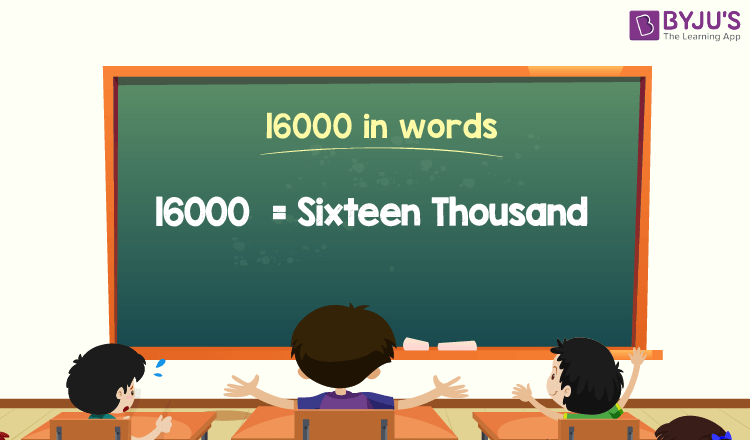# 16000 in Words

16000 in words is Sixteen Thousand. The number name of any number can be written using the ones, tens, hundreds, and thousands place of a number. Thus, the place value chart is helpful for writing the number 16000 in words. For example, if you earned Rs. 16000 in a month, you can write, “I have earned Rs. Sixteen Thousand in a month”.

 16000 in Words: Sixteen Thousand

In this article, let us discuss how to write 16000 in words, and look at the solved examples in detail.

## How to Write 16000 in Words?To write the number name of 16000, identify the place value of each digit of the given number 16000. Hence, for the number 16000, the digit in one’s place is 0, the digit in ten’s place is 0, the digit in hundred’s place is 0, and the number in thousand’s place is 16.

(i.e) 1’s place of 16000 is 0.

10’s place of 16000 is 0.

100’s place of 16000 is 0.

1000’s place of 16000 is 16.

Thus, the place value chart for 16000 is:

 Thousands Hundreds Tens Ones 16 0 0 0

Therefore, the number 16000 in words is Sixteen Thousand.

### Examples

Example 1:

Find the value of 20000 – 4000. Express the value in words.

Solution:

Given expression: 20000 – 4000

⇒ 20000 – 4000 = 16000

So, the value of 20000 – 4000 is 16000.

Hence, 16000 in words is sixteen thousand.

Example 2:

Express the value of sixteen thousand plus six thousand in words.

Solution:

Sixteen thousand = 16000

Six thousand = 6000

Sixteen thousand plus six thousand = 16000 + 6000 = 22000

Hence, the value of sixteen thousand plus six thousand is twenty-two thousand in words.

## Frequently Asked Questions on 16000 in Words

Q1

### Write 16000 in words.

16000 in words is sixteen thousand.

Q2

### Express the value of 16500 – 500 in words.

Simplifying 16500 – 500, we get 16000. Hence, 16000 in words is sixteen thousand.

Q3

### How to write sixteen thousand in numbers?

Sixteen thousand in numbers is 16000.

Q4

### Is the number 16000 an odd number?

No, the number 16000 is not an odd number.

Q5

### Is 16000 a prime number?

No, 16000 is not a prime number.